Related Articles
Sign Convention for Spherical Mirrors
• Last Updated : 17 Mar, 2021

While studying the reflection of light by spherical mirrors and the formation of images by spherical mirrors, a set of sign conventions are needed to learn that is required to measure the focal length, a distance of object or image from the mirror, and the magnification of the mirror.

Before, understanding the concept of sign conventions for spherical mirrors first discuss some commonly used terms in a spherical mirror.

### Commonly used terms in Spherical mirrors

1.  Aperture – The part of a spherical mirror that is exposed to all the light rays that incident on it is called the aperture of the spherical mirror. In other words, the diameter (XY) of the aperture of the concave and the convex mirror are shown in figure 1, known as its aperture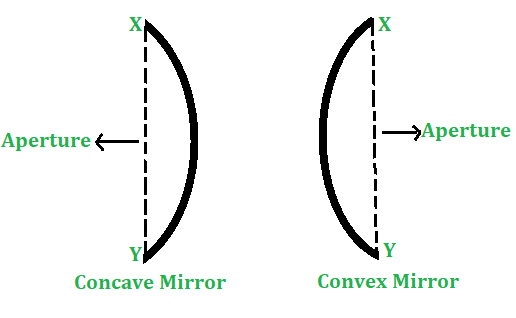Figure 1

2.  Center of curvature – The center of a hollow sphere of which the curved or spherical mirror forms a part is called the center of curvature. It is denoted by C (as shown in Figures 2 and 3).

3.  Radius of curvature – The radius of a hollow sphere of which the spherical mirror forms a part is called the radius of curvature. It is denoted by R

4.  Pole – The center of the spherical surface is called its pole. It is denoted by P

5.  Principal axis – The line joining the center of curvature (C) and pole (P) of a spherical mirror and extended on either side is called the principal axis.

6.   Principal focus – A point on the principal axis of a spherical mirror where the rays of light parallel to the principal axis meet or appear to meet after reflection from the spherical mirror is called the principal focus. It is denoted by F.

• In case of a concave mirror, the rays of light parallel to the principal axis after reflection actually meet the principal axis at F as shown in figure 2. So, the principal focus of a concave mirror is real.
• In case of a convex mirror, the rays of light parallel to the principal axis after reflection appear to meet or diverge from the principal axis at F as shown in figure 3. So, the principal focus of a convex mirror is virtual.

7.  Focal plane – A plane normal or perpendicular to the principal axis and passing through the principal focus (F) of a spherical mirror is called the focal plane of the spherical mirror.

8.  Focal length – The distance between the pole (P) and principal focus (F) of a spherical mirror is called the focal length of the mirror. It is denoted by f. In the figure 2 & 3 the focal length of the mirror is represented by PF.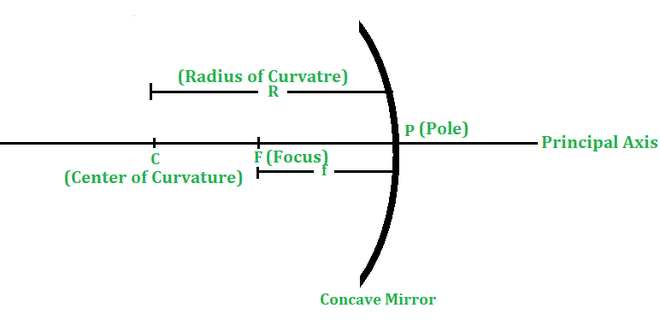Figure 2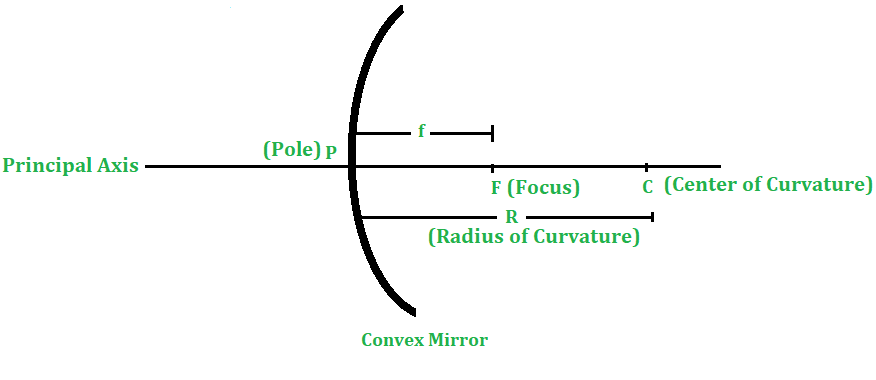Figure 3

### Sign Convention for spherical mirrors

The set of guidelines to set signs for image distance, object distance, focal length, etc for mathematical calculation during an image formation is called the Sign Convention. The sign conventions in case of the spherical mirrors are made in taking consideration that the objects are always placed on the left side of the mirror, such that the direction of incident light is from left to right. The sign conventions followed for any spherical mirror are given as:

1) All distances are measured from the pole of a spherical error.

2) Distances measured in the direction of incident light are taken as positive, while distances measured in a direction opposite to the direction of the incident light are taken as negative.

3) The upward distances perpendicular to the principal axis are taken as positive, while the downward distances perpendicular to the principal axis are taken as negative.

• For convenience, the object is assumed to be placed on the left side of a mirror. Hence, the distance of an object from the pole of a spherical mirror is taken as negative.
• Since the incident light always goes from left to right, all the distances measured from the pole (P) of mirror to the right side will be considered positive (because they will be in the same directions the incident light). On the other hand, all the distances measured from pole (P) of mirror to the left  will be negative (because they are measured against the direction of incident light)

### Important points to remember:

• According to the sign convention, the distances towards the left of the mirror are negative. Since as object is always placed to the left side of a mirror, therefore, the object distance (u) is always negative.
• The images formed by a concave mirror can be either behind the mirror (virtual) or in front of the mirror (real). So, the image distance () for a concave mirror can be either positive or negative depending on the position of the image.
• If the image is formed behind a concave mirror, the image distance (v) is positive but if the image is formed in front of the mirror , then the image distance will be negative.
• In a convex mirror, the image is always formed on the right-hand side (behind the mirror), so the image distance (o) for a convex mirror will be always positive.
• The focus of concave mirror is in front of the mirror on the left side, so the focal length of a concave mirror will be negative (and written with a minus sign, say, -10 cm).
• On the other hand, the focus of the convex mirror is behind the mirror on the right side, so the focal length (and written with a plus sign, say +20 cm or just 20 cm), a convex mirror is positive.
• The Focal Length and radius of curvature of a concave mirror are taken negative.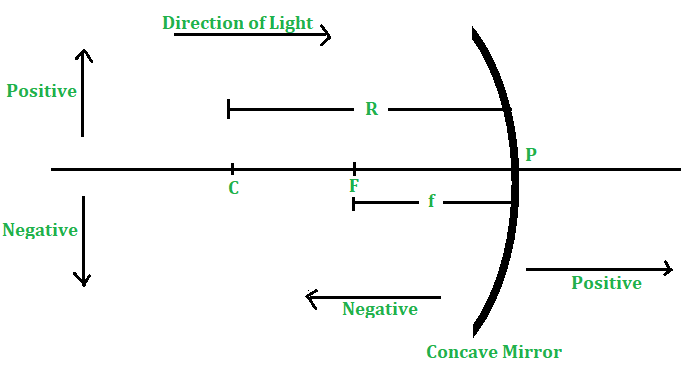Figure 4 -Concave Mirror

• The Focal Length and radius of curvature of a convex mirror are taken positive.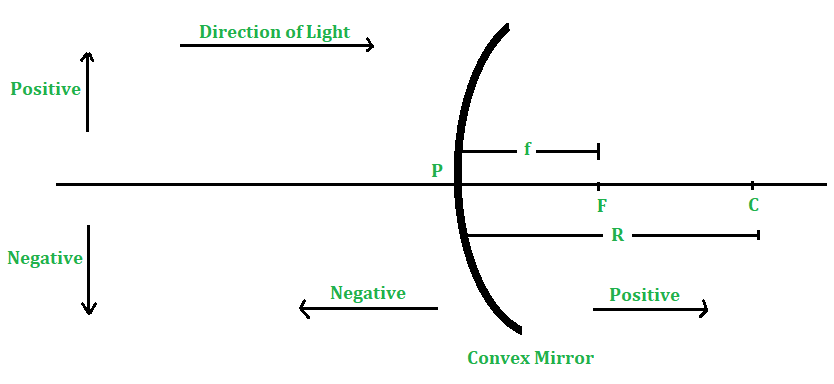FigureConvex Mirror

### Mirror Formula

The distance of the position of an object on the principal axis from the pole of a spherical mirror is known as object distance. It is denoted by u.

The distance of the position of the image of an object on the principal axis from the pole of a spherical mirror is known as image distance. It is denoted by v.

The relation between v and f of a spherical mirror is known as mirror formula.

It is given by

1/u + 1/v =1/f

or 1/object distance + 1/image distance = 1/ focal length

### Magnification (or Linear magnification)

Linear Magnification produced by a mirror is defined as the ratio of the size (or height) of the image to the size (or height) of the object. It is denoted by m.

If h’ = Size (or height) of the image produced by the mirror and h = Size (or height) of the object.

Then, linear magnification,

m = h/ h

or    m= height of image/ height of object

Linear magnification has no unit.

### Sample Problems

Example 1: A concave mirror produces two times magnified real image of an object placed at 10 cm in front of it. Find the position of the image.

Solution:

Here, -10 cm (Sign convention)

m=- 2 ( Image is real).

But m =-v/u

-2=-v/u

v=-20 cm

Thus, image of the object is at 20 cm from the pole of the mirror and in front of the mirror.

Example 2: An object of 5 cm in size is placed at a distance of 20 cm from it concave minor of focal length 15 cm At what distance from the mirror, should a screen be placed to get the sharp image? Also calculate the size of the image.

Solution:

Given that,

h=+5 cm

f=- 15.0 cm                              (Sign convention)

u=- 20 cm                               (Sign convention)

Determination of the position of image.

Using,

1/u + 1/v =1/f

We get ,

1/v = 1/f-1/u

v=-60cm

So the screen must be placed at a distance of 60 cm in front of the concave mirror.

Determination of size of the image and its nature.

Using,

m= h’/h =-v/u

h=-(v/u)h

hi=-15cm

Thus, the size of image -15cm, negative sign with h’ shows that the image is real and inverted

Example 3: An object 4 cm high is placed at a distance of 6 cm in front of c concave mirror of focal length 12 cm Find the position, nature and size of the image formed.

Solution:

Sound: It is produced in a material medium by a vibrating source when a material median vibrates it pushes air near it this is called compression (region of high pressure) and when the particle moves backwards it creates a region of low pressure known as rarefaction. due to this compression and rarefaction, sound waves travel. Sound waves required a median to travel material that can be the solid-liquid or the sound speed of sound is highest in solid.

Sound waves are longitudinal mechanical waves.

Sound waves can be divided into 3 categories:

1) Audible sounds:

The frequency of audible sound is between 20hz-20khz the human ear can hear this sound.

2.INFRASONIC SOUNDS:

these sound have a frequency of less than 20kz. Elephants and whales can hear this sound

3. Ultrasonic waves

these have a frequency greater than 20khz we cannot detect these sounds built the creatures like dogs, cats bats and mosquitos can hear it.

When a sound wave reaches our ear it is characterised by 3 factors:

1 pitch and frequency

2 loudness and intensity

3 quality

1. Pitch :
• It is thee characteristic of sound which distinguishes a shrilled soundwave from a dull or flat sound.
• The higher the frequency the higher is the pitch and the lower the frequency lower is their pitch.
• Frequency is defined as the number of cycles per unit of time.

2.Loudness:

It relates with the amplitude of the sound higher the amplitude higher is the loudness and lower the amplitude lower is the loudness.]

The intensity of sound at any point is defined as the amount of energy passing normally per unit area helped around that point per unit time si unit of intensity are watt/m2.

The intensity of sound at any point:

a ) Inversely proportional to the square of the distance of a point from the source.]

b)  directly proportional to the square of the amplitude, sq of frequency and density of the medium.

`I1/I2 = A21/A2 2`

Unit of loudness is bel

the practical unit of loudness is decibel.

`1db = 1/10 bel`

3. Quality: Quality in that characteristic of sound distinguishes between sound produced by two sources having the same intensity and pitch.

the quality of sound wave depends on factors like frequency and relative intensity of over tunes.zStep 1.

Here size of object, 4 cm

u=-6 cm (sign convention)

f=-12cm (sign convention)

Using, 1/u + 1/v =1/f

We get, Using,

-1/6 + 1/v =-1/12 ]

v=12cm

Image is formed at a distance of 12 cm  behind the concave mirror as v is positive. Therefore , image is virtual.

Using,  m= h’/h =-v/u

h’=(-12/-6)4

hi=8 cm

Example 4: A convex mirror used in a bus has radius of curvature 3.5 m. If the driver of  the bus locates a car at 10 m behind the bus, find the position, nature and size of the image of the car.

Solution:

Here, R = 3-5 m f = R 2 3-5 2 = 1.75m, u = – 100 m.

Determination of the position of the car.

Using, Using, 1/u + 1/v =1/f

1/v =1/f -1/u

1/v= 1/1.75 -1/(-10)

v=1.5m

Thus, the car appears to be at 1.5 m from the convex mirror and behind the mirror.

Determination of the size and nature of the image

Using, m= h’/h =-v/u

m=-1.5/-10

m=0.15

Thus, the size of the image of the car is 0.15 times the actual size of the car.

Since m is positive, so image of the car is virtual and erect (i.e., upright).

My Personal Notes arrow_drop_up